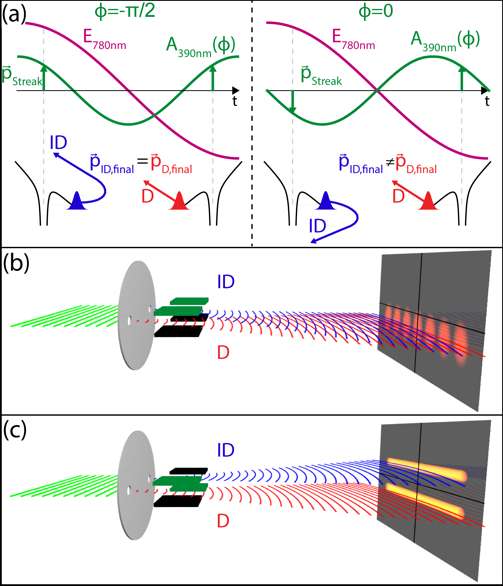## Streaking Temporal Double-Slit Interference by an Orthogonal Two-Color Laser Field

 When indistuingishable electron wave packets are launched from a sample at different times interferences occur in the final electron momentum distribution. Any which-way information will destroy the interference. The which-way information can either be stored in another particle by entanglement of the electron with the particle or in the electron itself, more precisely in a spin degree of freedom or in a motional degree of freedom like one of the momentum components. The interfering electron wave packets are launched by strong-field tunnel ionization whereby the most prominent interference fringes are the equidistant peaks in the electron energy distribution. These above-threshold ionization (ATI) peaks result from the interference of wave packets born periodically in time at subsequent laser cycles. 2005 additional fringes were discovered resulting from the interference between wave packets released within one cycle at times where the vector potential is the same but the direction of the electric field is opposite. In this work we show that orthogonally polarized two-color pulses can be used to turn these typically faint intracycle interference fringes into a dominating structure in the electron momentum distribution and, at the same time, can be used as a controllable which way marker.Figure 1: The vector potential of the second harmonic field relative to the ionizing 780 nm electric field and the electron trajectories ID (indirect) and D (direct) at two different phases between the colors. Though the fields are perpendicularly polarized in the experiment, here they are drawn in parallel for a more intuitive understanding of the streaking dynamics. For Φ=-π/2 both trajectories are streaked in the same direction allowing them to interfere in momentum space. For the phase Φ=0 trajectories ID and D are streaked to opposite directions which extinguishes the interference. (b) is a sketched spatial analogue where a double slit (depicted with two holes in a disk) transforms an incoming plane wave into two coherent spherical waves (for the sake of simplicity shown with curved lines). These waves are then steered into the same direction by two plane deflectors (capacitors) of equal polarity resulting in an interference pattern. This corresponds to the phase Φ=-π/2. (c) is the case for Φ=0 where the two waves are deflected in opposite directions showing no interference.Figure 2: Electron momentum distribution from strong-field single ionization of Argon. The data are integrated over an angular range ϑ = 90 ± 15° where ϑ = acos(px/√(px2+py2+pz2)) is the angle between the electron momentum vector and the normal to the (pz,py) plane. (a) Experiment with 780 nm (1.4⋅1014 W/cm2, 40 fs) pulse only. The laser polarization direction is shown by an arrow. (b) 780 nm / 390 nm orthogonal two-color pulse with an intensity ratio I390/I780 = 0.09 and a phase difference Φ = -π/2. The polarizations of the 780 nm and 390 nm lights are shown by red and blue arrows, respectively. The field driven momentum p = -A(t0) is shown by the dashed line. The finger-like structure results from the intracycle interference. (c) QMTC calculation for same laser parameters as in (b). Please check out PRL 114, 143001 (2015) for further information. Streaking Temporal Double-Slit Interference by an Orthogonal Two-Color Laser Field Martin Richter, Maksim Kunitski, Markus Schöffler, Till Jahnke, Lothar P. H. Schmidt, Min Li, Yunquan Liu, and Reinhard Dörner Physical Review Letter 114, 143001 (2015) Richter2015PRL.pdf